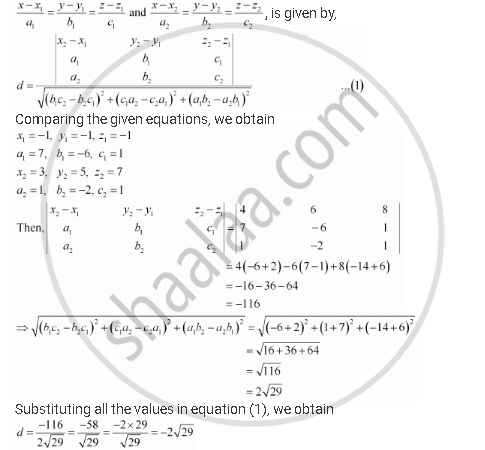Share

# Find the Shortest Distance Between the Lines (X+1)/7 = (Y+1)/(-6) = (Z+1)/1 and (X-3)/1 = (Y-5)/(-2) = (Z-7)/1 - CBSE (Commerce) Class 12 - Mathematics

ConceptShortest Distance Between Two Lines

#### Question

Find the shortest distance between the lines (x+1)/7 = (y+1)/(-6) = (z+1)/1 and (x-3)/1 = (y-5)/(-2) = (z-7)/1

#### Solution

The given lines are (x+1)/7 = (y+1)/(-6) = (z+1)/1 and (x-3)/1 = (y-5)/(-2) = (z-7)/1

It is known that the shortest distance between the two lines,Since distance is always non-negative, the distance between the given lines is 2sqrt29 units.

Is there an error in this question or solution?

#### APPEARS IN

NCERT Solution for Mathematics Textbook for Class 12 (2018 to Current)
Chapter 11: Three Dimensional Geometry
Q: 15 | Page no. 478

#### Video TutorialsVIEW ALL 

Solution Find the Shortest Distance Between the Lines (X+1)/7 = (Y+1)/(-6) = (Z+1)/1 and (X-3)/1 = (Y-5)/(-2) = (Z-7)/1 Concept: Shortest Distance Between Two Lines.
S